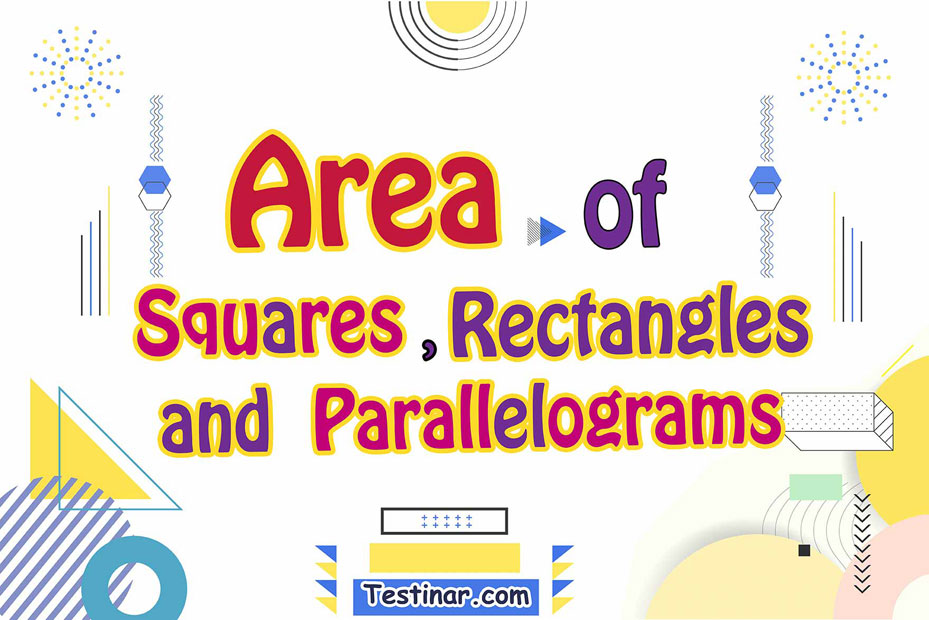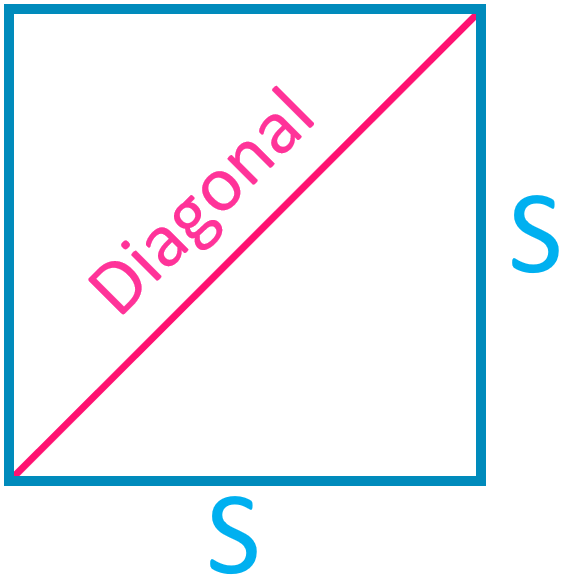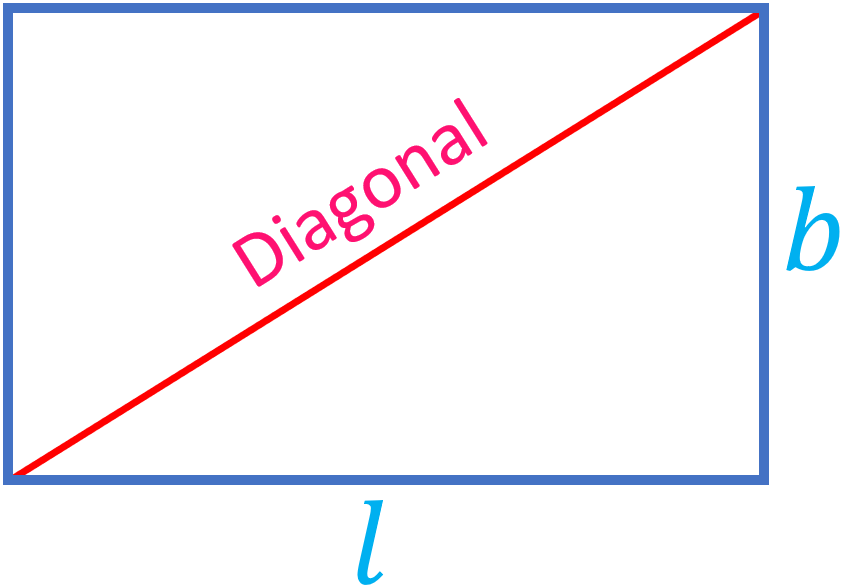## How to Find the Area of Squares, Rectangles, and Parallelograms

### What is the Parallelogram’s Area?

A parallelogram’s area is the space bounded by the parallelogram in a provided 2-dimension space. As stated earlier, a parallelogram is a particular kind of quadrilateral having $$4$$ sides, as well as the pair of opposite sides, are parallel. With a parallelogram, the opposite sides are an equal length and the opposite angles are also an equal measure. Because the rectangle and the parallelogram have properties that are similar, the rectangular’ s area equals the parallelogram’s area.

### Ways to Calculate a Parallelogram’s Area

Step one: Note down this formula: $$A \ = \ bh$$. $$A$$ stands for the area, while $$b$$ stands for the parallelogram’s length, and $$h$$ stands for the parallelogram’s height.
Step two: Find the parallelogram’s base. The base equals the length of the bottom side of a parallelogram.
Step three: Find the parallelogram’s height. The height is the length a perpendicular line has to go from the bottom to the top of a parallelogram.
Step four: Multiply the height and the base together.

### What is the Square’s Area?

Squares are closed 2-dimensional shapes having $$4$$ equal sides as well as $$4$$ equal angles. The square’s $$4$$ sides create the $$4$$ angles at the vertices. The total of the full length of the sides of a square is the perimeter, as well as the full space the square occupies, is the square’s area. It’s a quadrilateral with these properties.

• Its opposite sides are parallel.
• All $$4$$sides are the same.
• All the angles are $$90°$$.

The formula to determine the area of a square when the sides are provided is:
Area of a square $$= \ Side \times Side \ = \ s^2$$A square’s area may additionally be calculated via the assistance of the square’s diagonal. The formula utilized for finding a square’s area if the diagonal is provided is:
Area of a square using diagonals $$= \ \frac{Diagonal^2}{2}$$

### What is the Rectangle’s area?

The area of a rectangle is calculated via the sides. Usually, a rectangle’s area equals the product of its length and width. The rectangle’s perimeter, though, equals the total of all its $$4$$ sides. So, the rectangle’s area is described as the area encompassed by the perimeter. Rectangles are quadrilaterals whose opposite sides are equal and parallel to one another. Because rectangles have $$4$$ sides, there are also $$4$$ angles. All the rectangle’s angles equal $$90$$ degrees; so, all the rectangle’s angles are right angles.

### Formula for Determining the Area of a Rectangle

A rectangle’s area is determined in units via multiplying the width (or breadth) by the rectangle’s length.
Area of a Rectangle $$A \ = \ l \times b$$Follow these steps to determine the area:

• Step one:  Write down the length and width dimensions using provided data
• Step two: Multiply the values of the length and width
• Step three: Write down the solution in square units

### Exercises for Area of Squares, Rectangles, and Parallelograms

1)2)3)4)5)6)7)8)9)10)1) $$\ \color{red}{77 \ m}$$2) $$\ \color{red}{100 \ cm^2}$$3) $$\ \color{red}{143 \ cm^2}$$4) $$\ \color{red}{168 \ cm^2}$$5) $$\ \color{red}{437 \ cm^2}$$6) $$\ \color{red}{80 \ m^2}$$7) $$\ \color{red}{9 \ cm^2}$$8) $$\ \color{red}{16 \ cm^2}$$9) $$\ \color{red}{121 \ cm^2}$$10) $$\ \color{red}{460 \ cm^2}$$## Area of Squares, Rectangles, and Parallelograms Practice Quiz

### CLEP College Algebra Preparation 2020 – 2021

$21.99$16.99

### SAT Math Study Guide

$19.99$14.99

### Comprehensive Common Core Grade 8 Math Practice Book

$19.99$14.99

### TASC Math for Beginners

$24.99$14.99